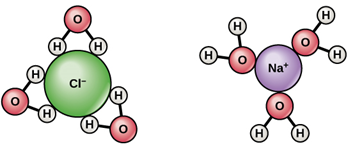# Hydration Enthalpy

## What is Hydration Enthalpy?

Hydration enthalpy (ꕔHHyd) is the change in enthalpy when one mole of gaseous ion under a standard condition of 1 bar pressure dissolves in a sufficient amount of water to form an infinitely dilute solution (infinite dilution means a further addition of solute will not cause any heat change).

In simple terms, enthalpy of hydration is described as the amount of energy released on dilution of one mole of gaseous ions. It can be considered as enthalpy of solvation with the solvent being water. Hydration enthalpy is also called hydration energy and its values are always negative.

For a chemical reaction,

M+(g) + aq → M+(aq) Enthalpy change = ∆HHyd

Water is considered to be a polar solvent because it has a positive (H atom) and negative (O atom) poles. When an ionic compound (any salt, say NaCl) is dissolved in water the solid-state structure of the compound is destroyed and the Na+ and Cl are separated.

In the solution, they exist as Na+ atoms surrounded by the negative ends of water molecules and similarly the Cl is surrounded by the positive end of water molecules as shown in figure (i). Since additional bonds are formed between the atoms the process releases some energy.

This energy is expressed as the hydration enthalpy or enthalpy of hydration between M+(g) and M+(aq) is that in M+(aq) the ion is surrounded by water molecules forming a weak bond.## Hydration Enthalpy of Elements

Hydration enthalpy values of various elements are tabulated in the table given below.

 Ion ꕔHHyd Li+ -520 Na+ -405 K+ -321 Rb+ -300 Cs+ -277 F- -506 Cl- -364 Br- -337 I- -296

The magnitude of hydration enthalpy depends on the charge density of the ions. The charge density is more for smaller ions and hence the smaller ions have higher values of hydration enthalpy. The higher the charge density the higher will be the force of attraction between the ion and the water polar end. This makes the value of hydration enthalpy higher in smaller ions. The alkali metals are highly hydrated, and the extent of hydration decreases down the group.

## Hydration Enthalpy and Solubility

The ions in a solute are bound together by coulombic force of attraction, to dissolve this solute into the solvent (here water) the water molecule should overcome this strong force of attraction. The energy required to break this string force of attraction is called lattice enthalpy.

Most of the ionic compounds are insoluble in non-aqueous solutions but they show high solubility in water. The factor that determines the solubility of a salt is the interactions of the ions with the solvent. As explained earlier the water is a polar molecule with a partial positive charge on hydrogen and partial negative charge on oxygen, interacts with the ions and forms a strong bond releasing energy.

The process of dissolution can be considered as a combination of two processes.

The first one is,

M+(s) → M+(g) ∆ = ∆HLatt Lattice enthalpy

The second process is the hydration,

M+(g) + aq → M+(aq) ∆ = ∆HHyd Hydration enthalpy

The first process involves the breaking of bonds in the solid solute; therefore, it is an endothermic process. Lattice enthalpy can be defined as the energy released when one mole ionic solid is converted gaseous ions. Greater the lattice enthalpy greater energy is required to overcome the force of attraction. Some compounds are insoluble in water because of their higher lattice enthalpy value.

The enthalpy of the solution. The dissolution process is dependent on both lattice enthalpy and hydration enthalpy. Enthalpy of the solution is the difference between hydration enthalpy and lattice enthalpy.

ie,

∆HSolution= ∆HHyd – ∆HLatt

∆HHyd = ∆HHyd(cation) + ∆HHyd

The enthalpy of the solution involves two processes, i.e., lattice energy and enthalpy of hydration.

The lattice energy of NaCl is the energy released when Na+ and Clions come close to each other to form a lattice.

NaCl(s) → Na+(g) +Cl(g) (I)

The enthalpy of hydration takes place when there is a dispersal of gaseous solute in water. It is the energy released when the solute transforms from a gaseous state to aqueous.

Na+(g) + Cl(g) → Na+(aq) + Cl(aq) (II)

On combining equations (I) and (II), the dissociation reaction of NaCl(s) into Na+(aq) and Cl(aq) is obtained as shown below

NaCl(s) → Na+(aq) + Cl(aq)

Therefore, the enthalpy of solution is calculated as:

ΔHsolution = Enthalpy of hydration – Lattice energy­­

Where,

• ΔHsolution is enthalpy of the solution.

Substitute the values in the above expression.

ΔHsolution = Enthalpy of hydration – Lattice energy = −783kJ mol-1 + 786kJ mol-1 = 3kJmol-1

The favourable conditions for the formation of the solution involve a negative value for ∆HSolution, ie, when the heat released on hydration is more than the heat required to overcome the force of attraction ie the lattice enthalpy.

## Application of Hydration Enthalpy

One application of enthalpy of hydration is the reaction of cement with water. The reaction being exothermic releases a large amount of heat. This heat released becomes significant in mass constructions like building dams and big structures. For the construction of massive concrete blocks, large quantities of cement are used.

During the process of setting the heat is released. The outer surfaces of the block cool relatively faster than the interior, this creates a thermal gradient in the block and can initiate cracks that lead to failure of the structure. To avoid this problem low heat types of cement are preferred for massive construction, cement with pozzolanic admixtures preferably fly ash or slag and also using ice instead of water to prepare concrete.

## Video Lesson – Heat of HydrogenationTest Your Knowledge On Hydration Enthalpy!